# Fraction calculator

The calculator performs basic and advanced operations with fractions, expressions with fractions combined with integers, decimals, and mixed numbers. It also shows detailed step-by-step information about the fraction calculation procedure. Solve problems with two, three, or more fractions and numbers in one expression.

## Result:

### 51/2 - 21/5 = 33/10 = 3 3/10 = 3.3

Spelled result in words is thirty-three tenths (or three and three tenths).

### How do you solve fractions step by step?

1. Conversion a mixed number 5 1/2 to a improper fraction: 5 1/2 = 5 1/2 = 5 · 2 + 1/2 = 10 + 1/2 = 11/2

To find new numerator:
a) Multiply the whole number 5 by the denominator 2. Whole number 5 equally 5 * 2/2 = 10/2
b) Add the answer from previous step 10 to the numerator 1. New numerator is 10 + 1 = 11
c) Write a previous answer (new numerator 11) over the denominator 2.

Five and one half is eleven halfs
2. Conversion a mixed number 2 1/5 to a improper fraction: 2 1/5 = 2 1/5 = 2 · 5 + 1/5 = 10 + 1/5 = 11/5

To find new numerator:
a) Multiply the whole number 2 by the denominator 5. Whole number 2 equally 2 * 5/5 = 10/5
b) Add the answer from previous step 10 to the numerator 1. New numerator is 10 + 1 = 11
c) Write a previous answer (new numerator 11) over the denominator 5.

Two and one fifth is eleven fifths
3. Subtract: 11/2 - 11/5 = 11 · 5/2 · 5 - 11 · 2/5 · 2 = 55/10 - 22/10 = 55 - 22/10 = 33/10
For adding, subtracting, and comparing fractions, it is suitable to adjust both fractions to a common (equal, identical) denominator. The common denominator you can calculate as the least common multiple of both denominators - LCM(2, 5) = 10. In practice, it is enough to find the common denominator (not necessarily the lowest) by multiplying the denominators: 2 × 5 = 10. In the next intermediate step, the fraction result cannot be further simplified by canceling.
In words - eleven halfs minus eleven fifths = thirty-three tenths.

#### Rules for expressions with fractions:

Fractions - use the slash “/” between the numerator and denominator, i.e., for five-hundredths, enter 5/100. If you are using mixed numbers, be sure to leave a single space between the whole and fraction part.
The slash separates the numerator (number above a fraction line) and denominator (number below).

Mixed numerals (mixed fractions or mixed numbers) write as non-zero integer separated by one space and fraction i.e., 1 2/3 (having the same sign). An example of a negative mixed fraction: -5 1/2.
Because slash is both signs for fraction line and division, we recommended use colon (:) as the operator of division fractions i.e., 1/2 : 3.

Decimals (decimal numbers) enter with a decimal point . and they are automatically converted to fractions - i.e. 1.45.

The colon : and slash / is the symbol of division. Can be used to divide mixed numbers 1 2/3 : 4 3/8 or can be used for write complex fractions i.e. 1/2 : 1/3.
An asterisk * or × is the symbol for multiplication.
Plus + is addition, minus sign - is subtraction and ()[] is mathematical parentheses.
The exponentiation/power symbol is ^ - for example: (7/8-4/5)^2 = (7/8-4/5)2

#### Examples:

subtracting fractions: 2/3 - 1/2
multiplying fractions: 7/8 * 3/9
dividing Fractions: 1/2 : 3/4
exponentiation of fraction: 3/5^3
fractional exponents: 16 ^ 1/2
adding fractions and mixed numbers: 8/5 + 6 2/7
dividing integer and fraction: 5 ÷ 1/2
complex fractions: 5/8 : 2 2/3
decimal to fraction: 0.625
Fraction to Decimal: 1/4
Fraction to Percent: 1/8 %
comparing fractions: 1/4 2/3
multiplying a fraction by a whole number: 6 * 3/4
square root of a fraction: sqrt(1/16)
reducing or simplifying the fraction (simplification) - dividing the numerator and denominator of a fraction by the same non-zero number - equivalent fraction: 4/22
expression with brackets: 1/3 * (1/2 - 3 3/8)
compound fraction: 3/4 of 5/7
fractions multiple: 2/3 of 3/5
divide to find the quotient: 3/5 ÷ 2/3

The calculator follows well-known rules for order of operations. The most common mnemonics for remembering this order of operations are:
PEMDAS - Parentheses, Exponents, Multiplication, Division, Addition, Subtraction.
BEDMAS - Brackets, Exponents, Division, Multiplication, Addition, Subtraction
BODMAS - Brackets, Of or Order, Division, Multiplication, Addition, Subtraction.
GEMDAS - Grouping Symbols - brackets (){}, Exponents, Multiplication, Division, Addition, Subtraction.
Be careful, always do multiplication and division before addition and subtraction. Some operators (+ and -) and (* and /) has the same priority and then must evaluate from left to right.

## Fractions in word problems:

• Sugar 8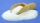Heather has 2 cups of powdered sugar. She sprinkles 3/5 of the sugar onto a plate of brownies and sprinkles the rest into a plate of lemon cookies. How much sugar does Heather sprinkle on the brownies? How much sugar does Heather sprinkle on the lemon coo
• Pizza fractionsAnn ate a third of a pizza and then another quater. Total part of pizza eaten by Ann and how much pizza is left?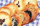In a cookie jar, 1/4 of the cookies are chocolate chip and 1/2 of the rest are peanut butter. What fraction of all the cookies are peanut butter?
• Magic bagEach time the prince crossed the bridge, the number of tolars in the magic bag doubled. But then the devil always conjured 300 tolars for him. When this happened for the third time, the prince had twice as much as he had in the beginning. How many tolars
• Farmer 5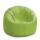Farmer Joe ordered 3 bags of soil last month. Each bag weighed 4 ⅖ kilograms. He used the first bag in a week. At the end of this month, there were 2 ¾ kilograms of soil left in the second bag and ⅞ kilograms of soil left in the third bag. How much soil w
• The chef 2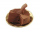The chef in the restaurant used 19/20  cup of chocolate chips and 1 1/10 cups of cocoa powder for the cake. How much more cocoa powder did the chef use than the chocolate chips?
• MartinMartin is making a model of a Native American canoe. He has 5 1/2 feet of wood. He uses 2 3/4 feet for the hull and 1 1/4 feet for a paddle. How much wood does he have left? Martin has feet of wood left.
• Package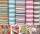The package was 23 meters of textile. The first day sold 12.3 meters. How many meters of textile remained in the package?
• Carrie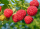Carrie picked 2/5 of the raspberries from the garden, and Robin picked some too.  When they were finished, 1/3 of the raspberries still needed to be picked.  What fraction of the raspberries did Robin pick? Use pictures, numbers or words and write your fi
• CurtainMrs. Lazo bought 9 1/8 m curtain cloth. She used 3 5/6 m to make a curtain for their bedroom. How many meters of cloth were not used?
• Difference of two fractions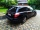What is the difference between 1/2 and 1/6? (Write the answer as a fraction in lowest terms. )
• Square metal sheetWe cut out four squares of 300 mm side from a square sheet metal plate with a side of 0,7 m. Express the fraction and the percentage of waste from the square metal sheet.
• Sum of 18Sum of two fractions is 4 3/7. If one of the fractions is 2 1/5 find the other one .